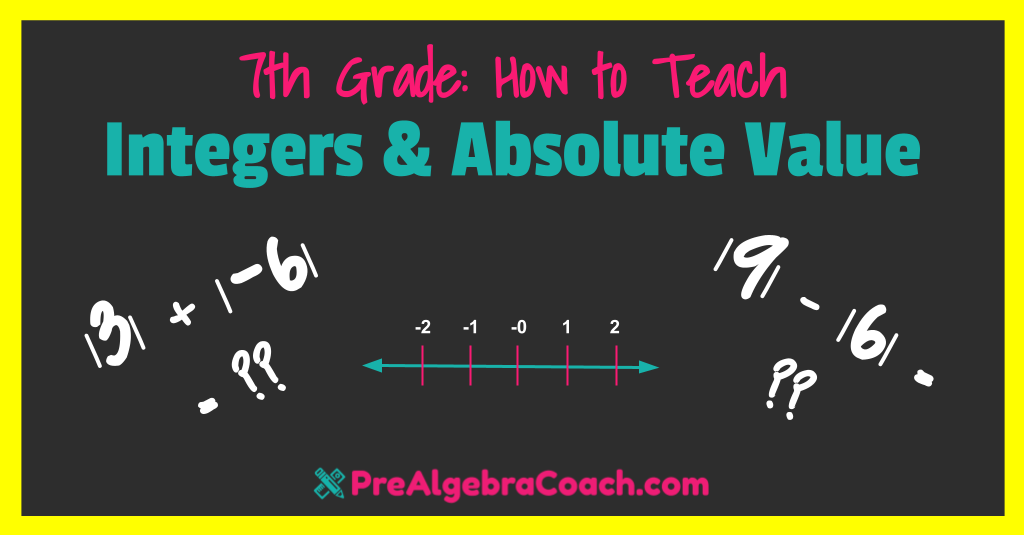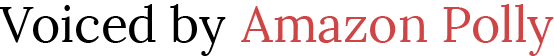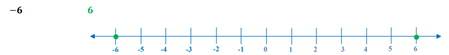# Teaching Integers and Absolute Value (7th Grade)

 Would You Rather Listen to the Lesson?In the 7th grade, children begin to understand the absolute value of integers and can interpret absolute value as magnitude for both positive and negative quantities in diverse real-life situations.

To help students achieve mastery of integers and absolute value (7th grade level), math teachers can prepare simple strategies for their lessons. We’ve compiled a list of several such strategies in this article, all of which will make teaching integers and absolute value an absolute breeze!

## Integers and Absolute Value (7th Grade) DefinitionWhen teaching about integers, define an integer as a whole number that can be positive or negative. Then, you can provide examples of integers, such as -38, -2, 25, 9, -12, etc. Also, check their knowledge of whole numbers by asking if some of the following numbers are integers: -1.54, 9.3, ⅓.

The absolute value of a number refers to the distance of that number from 0. Point out that we use one vertical bar on each side of the given number to represent absolute value. For instance, the absolute value of -5 is represented as |-5|.

Highlight that we can calculate the absolute value of both positive and negative integers and that negative integers are where things get interesting in terms of absolute value. Explain this with more practical examples, such as the ones in the following paragraphs.

## Teaching Integers and Absolute Value (7th Grade)

After providing the definitions of integers and absolute value, draw a big number line on the whiteboard to provide examples. Use markers with different colors, so that it’s even clearer. Now provide a few examples of graphing integers on the number line, such as:

• The temperature dropped 10 degrees below zero;
• An increase of 5 points;
• It was 31 feet below sea level;

Explain that each integer has an opposite integer, for instance, the opposite of -34 is +34 and the opposite of 125 is -125. Now use the number line to present this visually again, by asking students to graph an integer and its opposite on the number line.

For example, by asking them to graph -6 and its opposite, they’ll get the following:Now remind them of the definition of absolute value, that is, the absolute value of a given number is its distance from zero. Provide an example on the number line, for instance, ask children what the absolute value of 9 is.

By applying our definition, children will easily observe on the number line that the distance of 9 from 0 is 9. So the absolute value of 9 is 9, in other words, |9| = 9. Finding the absolute value of positive integers seems obvious, but as mentioned above, negative integers can be trickier.

To help students understand how to find the absolute value of negative integers, remind them that distance is always a positive quantity. This means that even if we’re asked to find the absolute value of -9, we’ll still have a positive quantity, that is, |-9| = 9.

Why? Well, according to our definition of absolute value, the absolute value of a number is the distance of that number from 0, so the distance from 0 to 9 is 9, but also, the distance from 0 to – 9 is 9 (we’ll move 9 spaces on the number line again). Use the number line to present this.

If you have the technical equipment in your classroom, you can also use this video by Khan Academy. It’s a great resource for explaining absolute value, as it contains colorful and engaging examples.

### Evaluating Expressions with Absolute Value

Once students are comfortable with graphing integers and finding the absolute values of positive and negative numbers, they can move on to more complex tasks, such as evaluating expressions with absolute value.

For example, show students how to evaluate the following expressions:

A) 54 – |+44| – |-8| =

54 – 44 – 8 =

10 – 8 =

2

B) |-24| – |8| x |-5| =
___
4

24 – 8 x 5 =
___
4

6 – 40 =
-34

## Activities to Practice Integers and Absolute Value (7th Grade)

### Absolute Value Millionaire Game

This online math game is a twist on the popular TV show ‘Who Wants to Be a Millionaire?’. Math Millionaire consists of several math questions related to integers and absolute value while offering multiple answers.

After answering a question correctly, students earn a money prize. The money prizes vary according to the difficulty of the problem. For example, for an easier question where children have to answer what is the absolute value of |-7|, they get 10\$, whereas for evaluating an expression such as |8 x (-7)|, they get 40\$.

To prepare for the game, make sure there are enough technical devices for all students in the classroom, such as computers, iPads, or other tablets. The game is played individually, which means that homeschooling parents can also use it to practice with their children.

### Absolute Value Matching GameThis is a simple game that requires very little prep work. You’ll need to create two sets of cards, and mark them with different colors, For example, if one set is green, the other one can be red.

Create math questions related to absolute value on one set and answers on the other set.

For example, if you have – | – 547 | in the red cards, make sure you have the solution in the green cards, that is – 547. Or if you have |½ – 20| in the red cards, you need to have 39/2 in the green ones. Include a mixture of easier and more challenging tasks.

1. Divide students into groups of 3 or 4.
2. Place the two piles of cards in the middle of each group. The cards should be facing downward.
3. Explain the rules of the game and tell children that each member of the group works closely with the others to match the green cards with the red cards.
4. After you’ve explained the rules of the game, children can open the cards and start playing.
5. The first group that manages to match all cards wins the game!
6. Play as many rounds as time allows or until children get tired.

If you’re a homeschooling parent, adjust the game by turning it into an individual game and simply place the sets of cards in front of your child and ask them to match them.

## Before You Leave…

If you’ve enjoyed these math strategies for teaching integers and absolute value, you’ll love our lesson that’s dedicated to this topic. So if you need guidance to structure your class and teach this, make sure to sign up for more free resources here!